An elk population $P(t)$ in a state park oscillates periodically as a function of time $t$ in months after January (so January is $t = 0$). In January, the population is at its lowest, with only $29$ members. The population is at its highest in July, with $43$ members.

(a) What is the period of the population $P(t)$?
period = months. help (numbers)

(b) What is the midline of the function $y=P(t)$?
$y =$ members. help (numbers)

(c) What is the amplitude of the function $P(t)$?
amplitude = members. help (numbers)

(d) Consider the six possible graphs of $P(t)$ below. Be sure to enlarge each graph and carefully read the labels on the axes in order distinguish the key features of each graph.

Which (if any) of the graphs A-F represents a possible graph of the population over two years?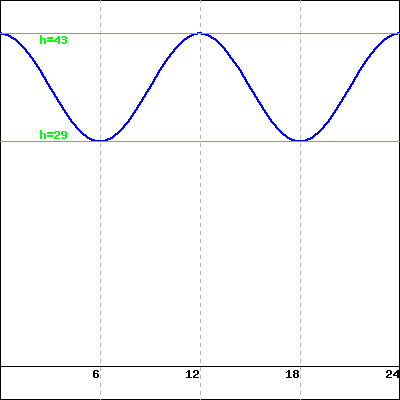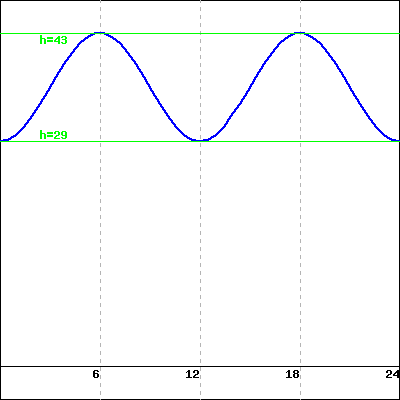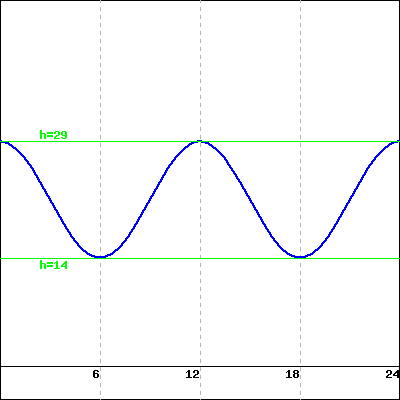A B C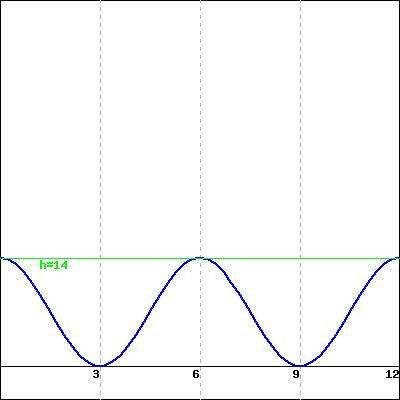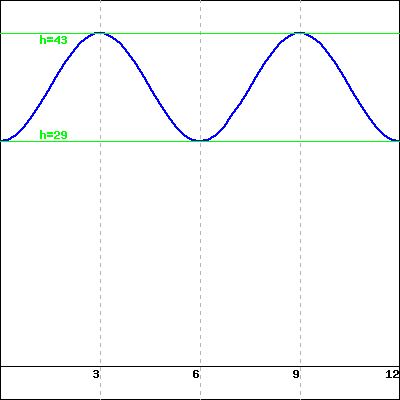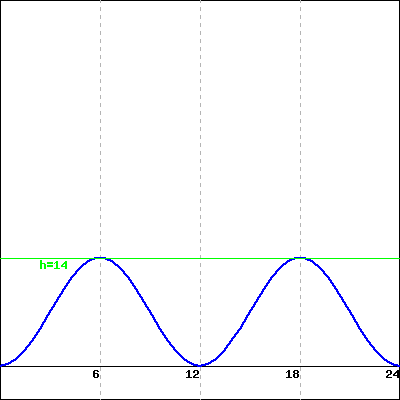D E F